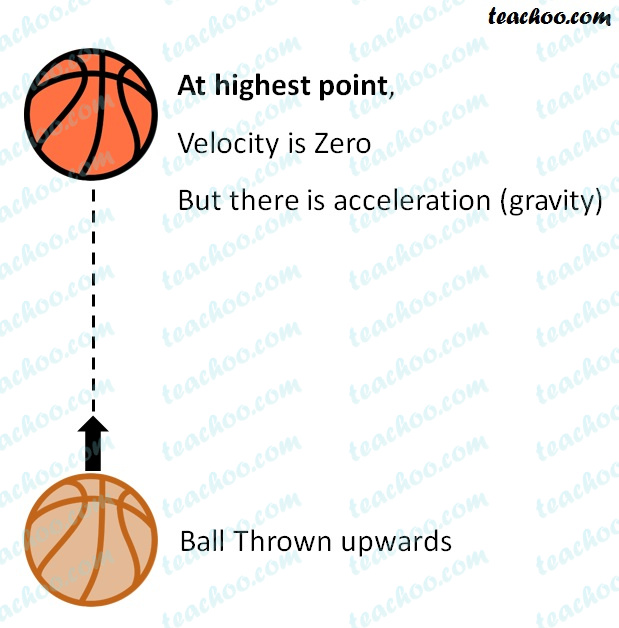NCERT Questions

Class 9
Chapter 8 Class 9 - Motion

## State which of the following situations are possible and give an example for each of these:

(a) an object with a constant acceleration but with zero velocity

When a body is at the highest point of its motion,

the velocity of the body becomes zero.

But it still accelerates with a constant acceleration,

which is equal to the acceleration due to gravity (g).

Thus, it is possible for a body to have zero

velocity and a constant acceleration.

####googletag.cmd.push(function() { googletag.display('div-gpt-ad-1669298377854-0'); });

(b) an object moving with an acceleration but with uniform speed.

In case of a uniform circular motion,

the direction of the motion is always

along the tangent.

Therefore, the speed is constant but

the velocity keeps changing as

the direction changes.

Acceleration is the rate of change of velocity. As the velocity is changing, an acceleration is produced.

Therefore, in uniform circular motion an object moves with an acceleration but with uniform speed.(c) an object moving in a certain direction with an acceleration in the perpendicular direction.

Whenever a body is in a uniform circular motion, the direction of the body is always perpendicular to the acceleration of the body.

The acceleration of the body is along the radius, while the direction of the body is along the tangent. Radius is perpendicular to tangent.

Thus, acceleration is perpendicular to the direction.

Learn in your speed, with individual attention - Teachoo Maths 1-on-1 Class